## Example Questions

### Example Question #16 : Decimals

Solve for: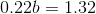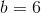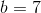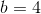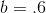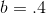Explanation:

To solve, first divide both sides of the equation by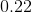, leaving you with: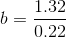.

If you have trouble dividing the decimals, you can multiply both of the numbers by one followed by a number of zeroes equal to the number of digits beyond the decimal point (one hundred, in this case), then divide, then divide your result by the same number (one hundred again).

### Example Question #17 : Decimals

Solve for(round to the nearest hundredth if necessary)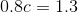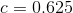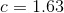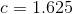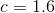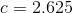Explanation:

To solve, first divide both sides of the equation by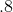: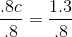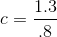To do this division, you can divide as normal, or if you are having trouble, you can multiply both numbers by 10 to eliminate the decimal: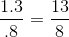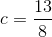### Example Question #18 : Decimals

Solve for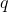: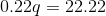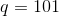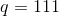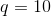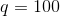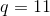Explanation:

To solve, first divide both sides of the equation by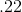so you isolate the variable: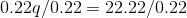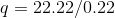Then, divide the decimal--if you have trouble doing so, remember that you can multiply both numbers byto eliminate the decimal, and then divide as normal: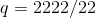### Example Question #19 : Decimals

Solve for: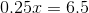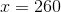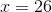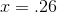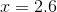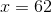Explanation:

To solve, first divide both sides of the equation by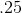so you can isolate the variable: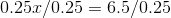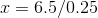Now divide.  If you have trouble dividing decimals, you may multiply both dividend and divisor byto eliminate the decimal and divide normally: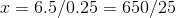### Example Question #11 : Decimal Operations

Solve for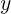: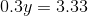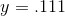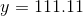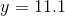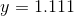Explanation:

To solve, first divide both sides of the equation by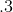so you isolate your variable: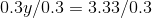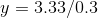Then, divide the decimals.  If you have trouble with this, you can multiply both numbers byto eliminate the decimals and then divide normally: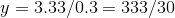Tired of practice problems?

Try live online GRE prep today.